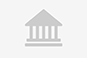# Prediction Maps & Validation using Logistic Regression & ROCTraining

Online

Descripción

• Tipología

Training

• Metodología

Online

• Horas lectivas

2h

• Inicio

Fechas disponibles

"In the this course,‑i have shared complete process‑(A to Z‑) based on my published articles, about‑how to evaluate and compare the results of applying the multivariate logistic regression method in Hazard prediction mapping using GIS and R environment.

Since last decade,‑geographic information system (GIS) has been‑facilitated the development of new machine learning, data-driven, and empirical methods that reduce generalization errors. Moreover, it gives new dimensions for the integrated research field.

STAY FOCUSED: Logistic regression (binary classification, whether dependent factor‑will occur (Y) in‑ a particular places, or not) used for fitting a regression curve, and it is a special case of linear regression when the output variable is categorical, where we are using a log of odds as the dependent variable.

Why logistic regression is special? It takes a linear combination of features and applies a nonlinear function (sigmoid) to it, so it’s a tiny instance of the neural network!

In the current course, I used experimental data that consist of : Independent factor Y (Landslide training data locations) 75 observations; Dependent factors X (Elevation, slope, NDVI, Curvature, and landcover)

I will explain the spatial correlation between; prediction factors, and the dependent factor. Also, how to find the‑autocorrelations between; the prediction factors, by considering their prediction importance or contribution. Finally, I will‑Produce susceptibility map using; R studio and ESRI ArcGIS‑only. Model prediction‑validation will be measured by most common statistical method of Area under (AUC) the‑ ROC‑curve.

At the the end of‑this course, you will be efficiently‑able to process, predict and validate‑any sort of data related to‑natural sciences‑hazard research, using advanced Logistic regression analysis capability.

Keywords: R studio, GIS, Logistic regression, Mapping, Prediction

"

Información importante

¿Qué objetivos tiene esta formación?: "Comprehensive understanding of Prediction Mapping Science and Tools in GIS
Validation using AUC of ROC Results of applying the multivariate logistic regression For Prediction Map
R-Code Script provided
My continuous support, taking your hand step-by-step to develop high quality prediction maps using real data"

¿Esta formación es para mi?: "Students and researchers of Natural hazards, environmental Science, Ecology, and Natural Sciences
Students and researchers interested in using GIS for producing hazard susceptible maps."

Requisitos: "No statistical background neededBasics background about ArcGIS software and RInterest in GIS prediction maps using real life Data"

Sedes y fechas disponibles

Ubicación

comienzo

Online

comienzo

Fechas disponibles Inscripciones abiertas

Opiniones

Materias

• ArcGIS
• ESRI
• GIS
• map
• model
• spatial
• Geographic
• Programming platform
• Programming Language
• Coding project
• WebGIS
• Web Programming
• R Language
• Language Programming
• Geospatial Data
• Geospatial concepts
• Coding
• Geomatics
• Geospatial
• GIS Data

### Temario

"Introduction
Introduction to Logistic Regression
Prepare dichotomous binary (1,0) training data in ArcGIS
Create dichotomous binary (1,0) training data
Merge binary dichotomous (0,1) training data
Settings and Packages preparation in R Studio
Working environment setting in R studio
Install multiple required packages
Export raster factors from ArcGIS into R studio
Data Visualization and preparation in R studio
Load and Plot raster factors in R
More about raster visualization and export in R
Classify Raster Factors In R
Raster breaks and color bars in R
Crop a Specific Raster Area In R
Data conversion and resampling in R Studio
Conversion of Dependent and Independent Factors for LR Model in R
Categorical Independent Factors for LR Model
Preparation of Categorical Independent Factor for LR Model
Data Resampling in R
Run multivariate Logistic Regression in R
Stacking Dependent and Independent Rasters in R
Remove No Data (NA) and Produce Data Frame Table
Run Logistic Regression Function
Run ANOVA and McFadden R squared Tests
Confusion Matrix of Prediction Results in R
ROC and Model Validation
Calculate and Plot AUC of ROC Curve for Validation Assessment
Graphing a Probability Curve S-Shape with Multiple Predictors
Exercise No. 1
Produce Prediction Index Map Using LR coefficients in R Studio
Produce Prediction Index Map Using LR coefficients in ArcGIS"

Prediction Maps & Validation using Logistic Regression & ROC